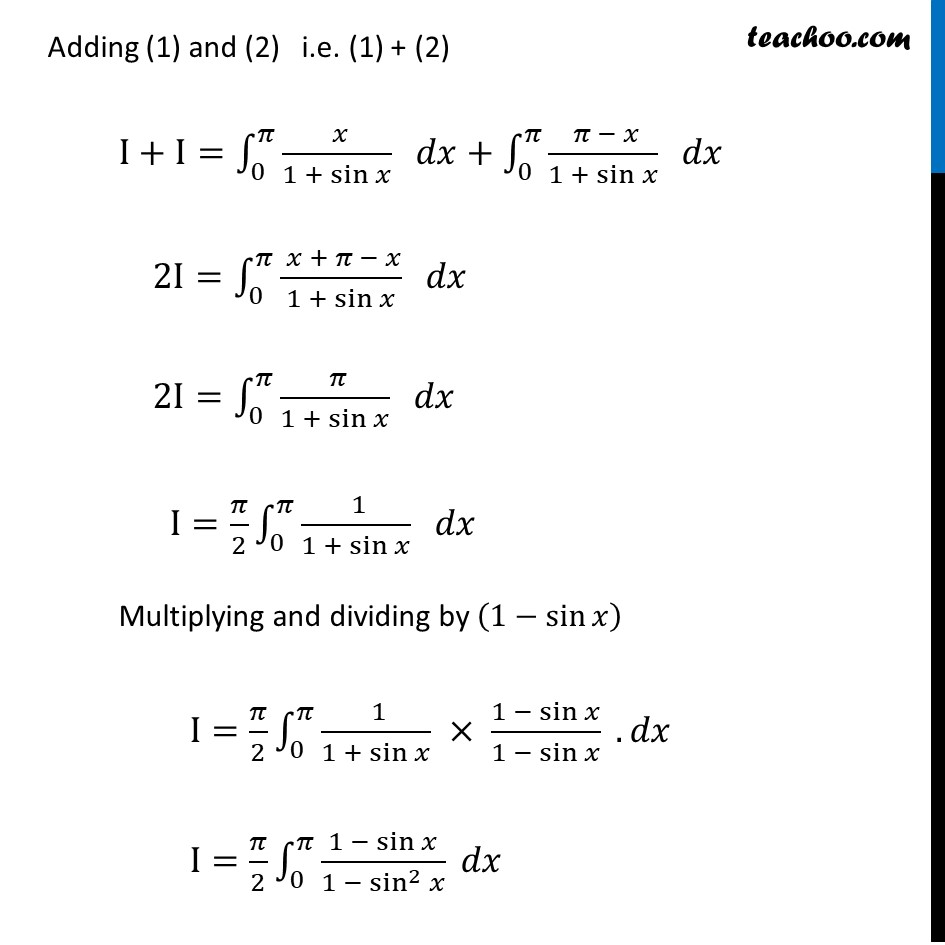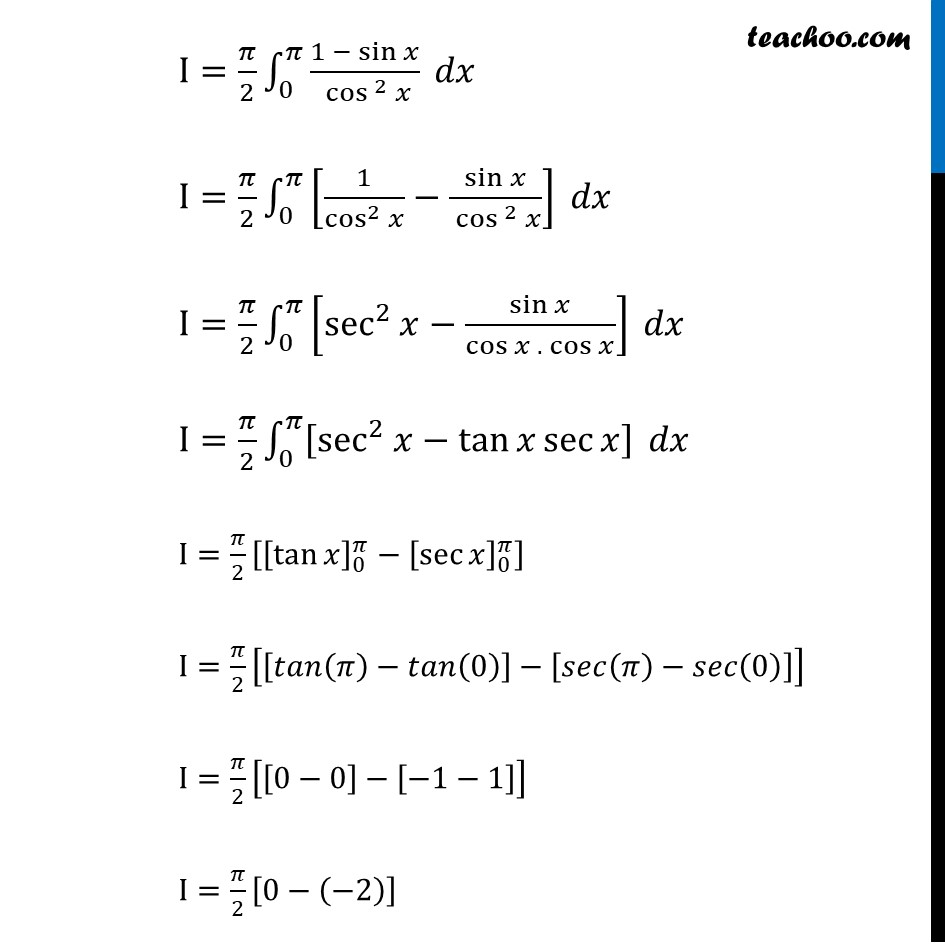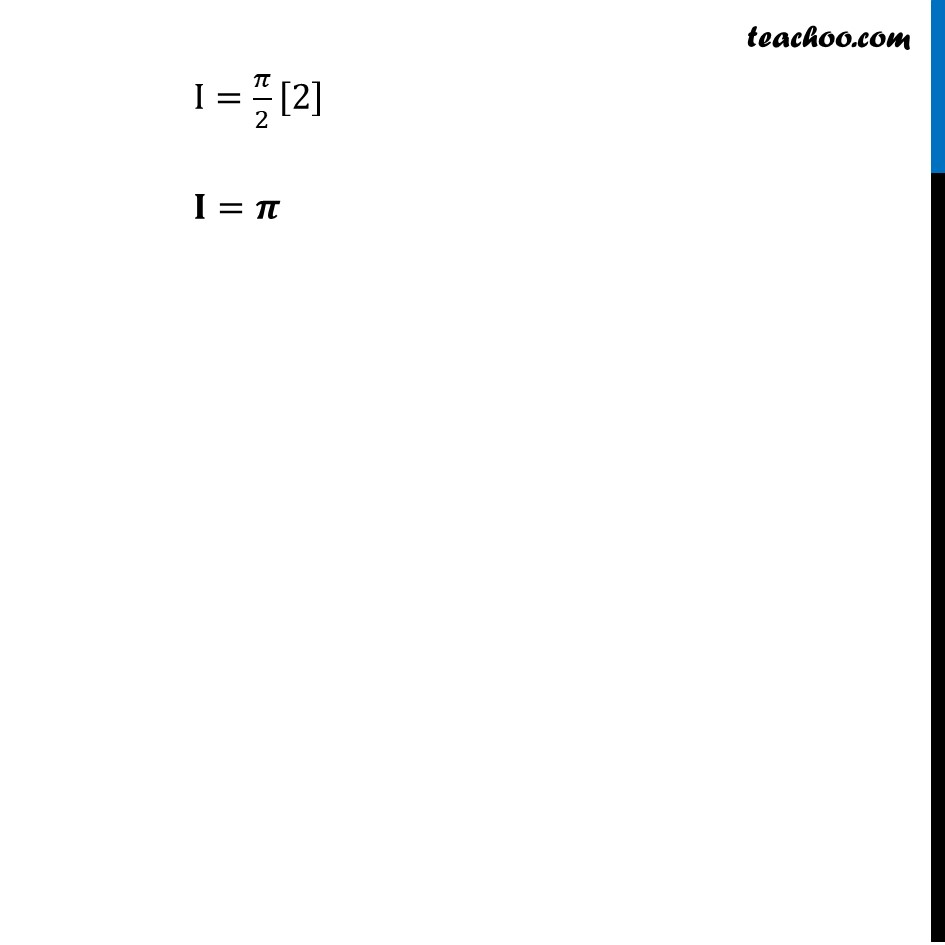Definite Integration by properties - P4

Chapter 7 Class 12 Integrals
Concept wiseLearn in your speed, with individual attention - Teachoo Maths 1-on-1 Class

### Transcript

Ex 7.10, 12 By using the properties of definite integrals, evaluate the integrals: ∫_0^𝜋▒(𝑥 𝑑𝑥)/(1 + sin⁡𝑥 ) 𝑑𝑥 Let I=∫_0^𝜋▒𝑥/(1+ sin⁡𝑥 ) 𝑑𝑥 ∴ I=∫_0^𝜋▒(𝜋 − 𝑥)/(1+ sin⁡𝑥 ) 𝑑𝑥 Adding (1) and (2) i.e. (1) + (2) I+I=∫_0^𝜋▒( 𝑥)/(1 + sin⁡𝑥 ) 𝑑𝑥+∫_0^𝜋▒( 𝜋 − 𝑥)/(1 + sin⁡𝑥 ) 𝑑𝑥 2I=∫_0^𝜋▒( 𝑥 + 𝜋 − 𝑥)/(1 + sin⁡𝑥 ) 𝑑𝑥 2I=∫_0^𝜋▒( 𝜋)/(1 + sin⁡𝑥 ) 𝑑𝑥 I=𝜋/2 ∫_0^𝜋▒( 1)/(1 + sin⁡𝑥 ) 𝑑𝑥 Multiplying and dividing by (1−sin⁡𝑥 ) I=𝜋/2 ∫_0^𝜋▒〖( 1)/(1 + sin⁡𝑥 ) × (1 − sin⁡𝑥)/(1 − sin⁡𝑥 )〗 . 𝑑𝑥 I=𝜋/2 ∫_0^𝜋▒(1 − sin⁡𝑥)/(1 − sin^2⁡𝑥 ) 𝑑𝑥 I=𝜋/2 ∫_0^𝜋▒(1 − sin⁡𝑥)/( 〖cos 〗^2⁡𝑥 ) 𝑑𝑥 I=𝜋/2 ∫_0^𝜋▒[1/cos^2⁡𝑥 −sin⁡𝑥/( 〖cos 〗^2⁡𝑥 )] 𝑑𝑥 I=𝜋/2 ∫_0^𝜋▒[sec^2⁡𝑥−sin⁡𝑥/(cos⁡𝑥 .〖 cos〗⁡𝑥 )] 𝑑𝑥 I=𝜋/2 ∫_0^𝜋▒[sec^2⁡𝑥−tan⁡〖𝑥 sec⁡𝑥 〗 ] 𝑑𝑥 I=𝜋/2 [[tan⁡𝑥 ]_0^𝜋−[sec⁡𝑥 ]_0^𝜋 ] I=𝜋/2 [[𝑡𝑎𝑛(𝜋)−𝑡𝑎𝑛(0)]−[𝑠𝑒𝑐(𝜋)−𝑠𝑒𝑐(0)]] I=𝜋/2 [[0−0]−[−1−1]] I=𝜋/2 [0−(−2)] I=𝜋/2  𝐈=𝝅